The Big E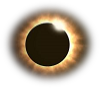The Solar Eclipse

Most of us have had the chance to observe the Solar Eclipse at some point in our lives. Even though we are told not to, who of us hasn't peeked up at the Sun just as the Moon passes directly in front of it? What we observe is the Moon perfectly blotting out the Sun, appearing as though the two were the same size, though we

know the Sun is significantly larger. Have you ever considered the facts that make this possible?Facts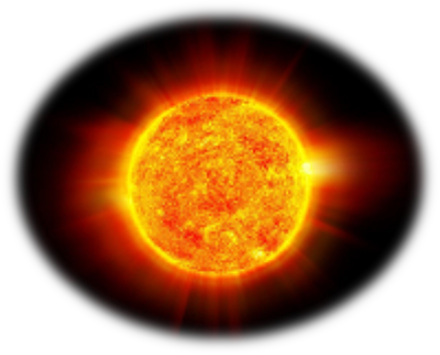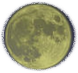In comparison, if the Moon were a 0.07 inch piece of sand, the Sun would be a 27 inch beach ball

The diameter of our Sun measures 1,377,648 km

The diameter of our Moon measures 3,476 km

By doing the math, we find the Sun is 396.3 times larger than the Moon

With the Sun being 396.3 times larger than the moon, what causes them to appear the same size?  Keeping that number in mind, we'll now look at their associated distances from Earth.

Orbital Distances

As the Earth orbits the Sun, it follows an elliptical path rather than a perfect circle. At its closest (Perigee), the Sun is 91.4 million miles (147.1 million km) away from us. At its farthest (Apogee), the Sun is 94.5 million miles (152.1 million km) away, giving us an average of 92.95 million miles (149.6 million kilometers).

Likewise, the Perigee of the Moon's orbit around Earth is 225,622 miles(363,104 km) while the Apogee is 252,709 miles (406,696 km). At a point just below the average in the orbit, we find a distance of 234,563 miles (377,492 km).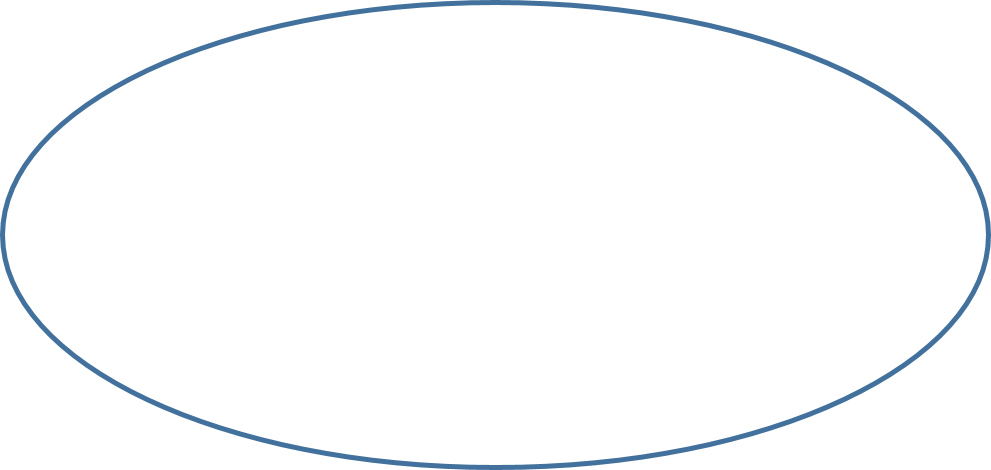377,492 km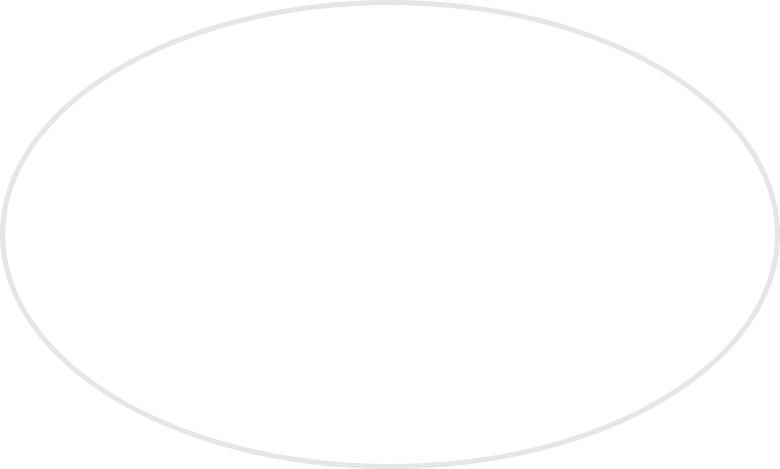149.6 million kilometers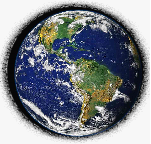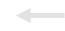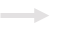*graph not to scale

By doing the math, we find the Sun is 396.3 times further from Earth than the Moon

More simply put, the Sun is approximately 400 times larger than the Moon, but is also 400 times further away.  This perfect ratio is what creates the 1:1 perspective we have from Earth..

Could this perfect ratio be only a result of chance? Or does it give evidence of intelligent design?

The heavens declare the glory of God; the skies proclaim the work of his hands. Psalms 19:1 (written ~ 1015 B.C.)